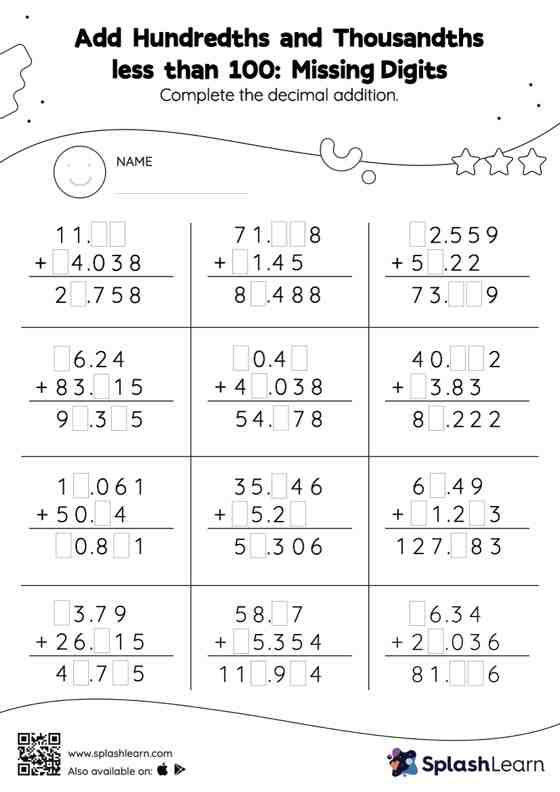# Add Hundredths and Thousandths less than 100: Missing Digits Worksheet

Home > Add Hundredths and Thousandths less than 100: Missing DigitsCan your young mathematician add hundredths and thousandths less than 100? That's exactly what they do here. Students align the decimal points and use zero as space holders to add decimals. Then they use the relationship between addition and subtraction to find the missing number in add hundredths and thousandths less than 100 worksheet. In this worksheet, numbers are laid one on top of another (vertical format). This encourages students to use the inherent place value structure to solve the problems.